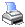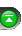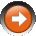﻿ CalculateOnBarClose
 NinjaScript > Language Reference > Data > CalculateOnBarCloseDefinition

 • When indicators or strategies are running on historical data, OnBarUpdate() is only called on the close of each historical bar even if this property is set to false. This is due to the fact that with a historical data set, only the OHLCVT of the bar is known and not each tick that made up the bar.

Property Value

This property returns true if the value is calculated on the close of each bar; otherwise, false. Default set to true.

Syntax

CalculateOnBarClose

Examples

 protected override void Initialize() {     // Calculate on the close of each bar     CalculateOnBarClose = true; }

Tips

 1 Calculating indicators or systems for each incoming tick can be CPU intensive. Only calculate indicators on each incoming tick if you have a requirement to calculate it intra-bar.
 2 For an example of how to separate some logic to be CalculateOnBarClose = true and other logic to be false please see this reference sample.
 3 Embedded indicators within a strategy should not use a CalculateOnBarClose property since it is already utilizing the CalculateOnBarClose property of the strategy.